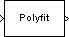# Least Squares Polynomial Fit

Compute polynomial coefficients that best fit input data in least-squares sense

## Library

Math Functions / Polynomial Functions

`dsppolyfun`

•## Description

The Least Squares Polynomial Fit block computes the coefficients of the nth order polynomial that best fits the input data in the least-squares sense, where you specify n in the Polynomial order parameter. A distinct set of n+1 coefficients is computed for each column of the M-by-N input, u.

For a given input column, the block computes the set of coefficients, c1, c2, ..., cn+1, that minimizes the quantity

`$\sum _{i=1}^{M}{\left({u}_{i}-{\stackrel{^}{u}}_{i}\right)}^{2}$`

where ui is the ith element in the input column, and

The values of the independent variable, x1, x2, ..., xM, are specified as a length-M vector by the Control points parameter. The same M control points are used for all N polynomial fits, and can be equally or unequally spaced. The equivalent MATLAB® code is shown below.

`c = polyfit(x,u,n) % Equivalent MATLAB code `

For convenience, the block treats length-M unoriented vector input as an M-by-1 matrix.

Each column of the (n+1)-by-N output matrix, c, represents a set of n+1 coefficients describing the best-fit polynomial for the corresponding column of the input. The coefficients in each column are arranged in order of descending exponents, c1, c2, ..., cn+1.

## Examples

In the ex_leastsquarespolyfit_ref model below, the Polynomial Evaluation block uses the second-order polynomial

`$y=-2{u}^{2}+3$`

to generate four values of dependent variable y from four values of independent variable u, received at the top port. The polynomial coefficients are supplied in the vector `[-2 0 3]` at the bottom port. Note that the coefficient of the first-order term is zero.The Control points parameter of the Least Squares Polynomial Fit block is configured with the same four values of independent variable u that are used as input to the Polynomial Evaluation block, `[1 2 3 4]`. The Least Squares Polynomial Fit block uses these values together with the input values of dependent variable y to reconstruct the original polynomial coefficients.

## Parameters

Control points

The values of the independent variable to which the data in each input column correspond. For an M-by-N input, this parameter must be a length-M vector. Tunable (Simulink).

Polynomial order

The order, n, of the polynomial to be used in constructing the best fit. The number of coefficients is n+1.

## Supported Data Types

• Double-precision floating point

• Single-precision floating point

 Detrend DSP System Toolbox Polynomial Evaluation DSP System Toolbox Polynomial Stability Test DSP System Toolbox `polyfit` MATLAB